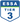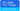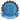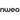Pranalika Mahanta

All Posts

Math can be tricky sometimes, especially when we are solving problems. These tricky situations tied up with the need for agility to solve and learn new concepts make it even harder for students who lack basic solving abilities.

According to the Huffpost, the three most common reasons why students lack basic mathematical skills are:

1. Not seeking help from their teachers
2. Not understanding the terms and symbols in the class
3. Not practising math problems on a regular basis

Although we know how to tackle most of these issues as teachers, we don’t exactly do it in an effective and attention mongering way. This leads to more confusion and questions in the student’s mind.

To overcome these challenges and also to make the lessons more effective, use these 5 math tricks that should help your students in solving problems faster.

## 1. Count from the number method to add numbers

For example, if you want to solve 5 + 3…• Start on 5
• Count the number you want to add (in this case, 3)

## 2. Draw & Use the Multiplication Table

The best way to multiply any number with the other is to use multiplication tables. For example, if you want to solve 8 x 2…• Draw a table as is or download the printable version from here.
• Lookup for the first number you want to multiply. In this case, pick 8 from either the row or column in the table.
• Now, look for the intersection of other numbers table. In this case, pick 2 from the other row or column based on what was selected previously.

## 3. The Subtraction Method to multiply any number by 9

This is one of the most familiar tricks known to all. In this instance, let’s solve 9 x 3…

• Subtract 1 from the number by which you are multiplying the number 9. In this case, 3 – 1 = 2. So, 2 is the first number in your answer.
• Now subtract 9 from the answer you got above. In this case, 9 – 2 = 7. So, 7 is the second number in your answer.
• So the answer is 27.

## 4. Finding 5% Of Any Number Using the Decimal Method

Finding the percentage of any number is very easy following this method. Here, let’s find out 5% of 235…

• Move the decimal point after one digit. In this case, here 235 will become 23.5
• Divide the answer you got by 2. Here, 23.5 / 2 = 11.75 which should be the answer to the original question.

## 5. Multiplying Numbers That End With Zero (0) Using Retain Zero Method

Multiplying any number with zeros, in the end, can easily be solved. Let’s take an example of 200 x 400…

• Remove all the zeros towards the end and multiply the numbers. Here, 200 x 400 will become 2 x 4 = 8
• Now add the removed zeros to the answer. In this case, the answer is 8, so the final answer will be 80,000 (where 4 zeros retained are added back).

Using these tricks not only helps your students in understanding the lessons better and solving problems faster but also makes your students more confident in taking up new problems and lessons.

In addition to these tricks, you can also introduce Prodigy which will help in making your lessons more effective and presentable to the students.

••••